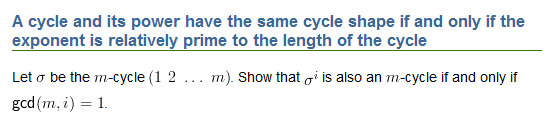# Questions on the symmetric group

Maths Lover
first ,
if p is prime , show that an element has order p in Sn iff it's cycle decomosition is a product of commuting p-cycles

my solution is very diffrent about the one in the book and I don't know if my strategy is right

my proof
______
let T is an element of Sn
and the cycle decomposition of T = t1 t2 t3 ... tm
t1 , t2 , ... , tm are disjoint cycle
so
ti tj = tj ti for all i,j
T^p = ( t1 t2 ... tm )^p = (t1)^p (t2)^p ... (tm)^p
now , ti , tj are 2 disjoint cycle so if ti or tj are not equal to identity permutation then ,
ti tj =\= e

now if T^p = 1
then
(t1)^p (t2)^p ... (tm)^p = 1
but (t1)^p , (t2)^p , ... , (tm)^p are disjoint
so if (ti)^p =\= 1 for all i then
(t1)^p (t2)^p ... (tm)^p =\= 1
but (t1)^p (t2)^p ... (tm)^p = 1
so it's nessary that
(ti)^p = 1 for all i
so
l ti l = p
and ti 's are commuting because they are disjoint

so we proved that order of T = p where p is prime then it's cycle decomposition has the same order p
now
every t1 is a p-cycle

is this right ?

the otherway is easy to prove

in this question we can't use the rule which says @ order of an element in Sn is the LCD of the lenghts of the cycles in its cycle decomposition because this rule didn't come in the book and I search for a proof without it

Maths Lover
here is the question on more clear way

prove that :Homework Helper
Gold Member
2022 Award
On the face of it, that's a rather different question (though no doubt they're equivalent in some way). So if the second post is the real question to be answered, please repost your proof in a way that relates more directly to it.

Maths Lover
On the face of it, that's a rather different question (though no doubt they're equivalent in some way). So if the second post is the real question to be answered, please repost your proof in a way that relates more directly to it.

sorry , a mistake was made !
my question is the first one ,
the second one I work on it

so , does my attempt on the first question is right or not ?!

Homework Helper
Gold Member
2022 Award
if T^p = 1
then
(t1)^p (t2)^p ... (tm)^p = 1
Not immediately. Tp = ((t1) (t2) ... (tm))p = 1. You need to use the fact that (t1) , (t2) , ... , (tm) are disjoint to show they commute.

Maths Lover
Not immediately. Tp = ((t1) (t2) ... (tm))p = 1. You need to use the fact that (t1) , (t2) , ... , (tm) are disjoint to show they commute.

yes , I said that t1 , t2 , ... , tm are disjoint beacuse t's are the cycle decomposition
so they are disjoint so they are commuting with each other !